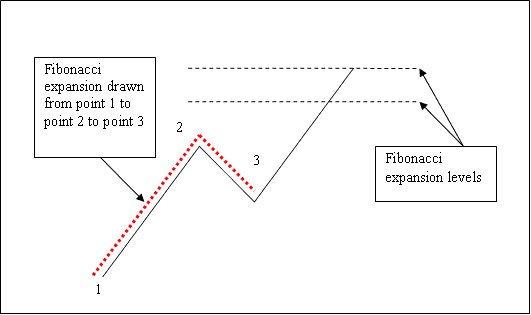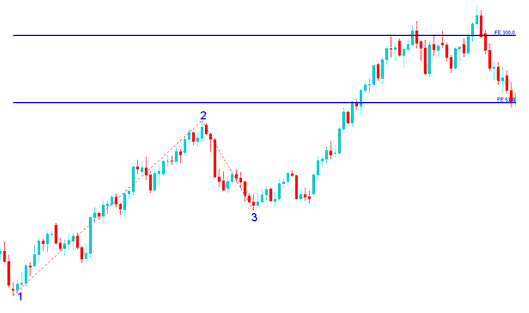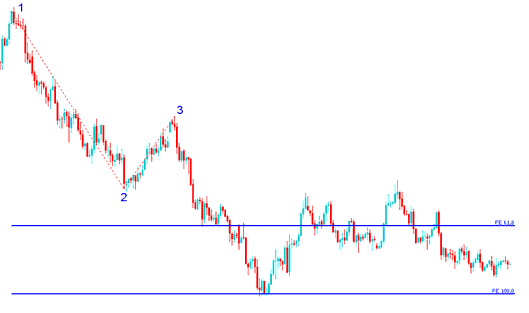# Stock Indices Fibonacci Expansion Levels

We have looked at Fibonacci retracement in the previous lesson, drawn between 2 points. But to draw Fibonacci expansion we use 3 chart points.

To draw these levels we wait until the retracement is complete and the stock indices instrument starts to move in the original direction of the Stock Indices trend. Where the retracement reaches is used as point 3.

The example illustrated below shows the 3 Points where the Fibonacci extension is drawn, marked as 1, 2 and 3. 1 is where the stock indices trend started, 2 is where the stock indices trend pulled back and retraced and 3 is where the retracement reached as shown on the stock indices example illustrated below.Please note where these levels are drawn - they are drawn above the indicator, these are the points where the stock indices trader will set the take profit orders.

### Drawing Expansion Levels on an Upward Trend

We use Fibonacci extension levels to estimate where the movement will reach. There are 2 important extension levels; 61.8 % and 100 %, these are used for taking profit.

On the example, below you can see that the Fibonacci extension is drawn along the direction of the trend, since the stock indices trend is upward - the expansion is drawn upwards.

These levels are displayed as horizontal lines above the indicator, showing profit taking areas. In the stock indices example illustrated below if you had used of 100 % extension you would have made nice profit from the trade.

NB: This is the same trade from the previous example where we used Fibonacci retracement to buy at around retracement level 38.2 %. At the same time we have used Fibonacci expansion 100 % to set take profit. Now find a Stock Indices Trading chart and practice these strategies.From the above examples, the upward stock indices trend continued and both 61.8 % and 100.0 % levels were all hit after which stock index price retraced again after hitting the 100.0 % extension.

### Drawing Expansion Levels on a Downward Trend

Since we use this tool to estimate take profits, how do we draw it in a downward Indices trend?

We draw it from point 1 to 2 to 3 as shown below. Remember we always draw this tool in the direction of the trend. In the stock indices example illustrated below, can you figure what direction we have drawn it? That is right - downwards.

Try and see the difference between how we have drawn it above and how it is drawn below. This time you would also have used extension level 100 %, see just where the stock index price reached. That would have been a nice take profit area.From the stock indices example above, after plotting this tool there are two levels that are used to show the profit taking areas, these two are drawn as horizontal lines across the stock index price chart.

### Stock Index Symbol

S&P500, Nasdaq100, Dow Jones Industrial Average 30 - DJI30, Nikkei225, BSE SENSEX, MSCI World, Nasdaq Composite, DAX PERFORMANCE-INDEX, FTSE100 Index, Hang Seng Index, KOSPI Composite Index, CAC40, IBOVESPA, Hang Seng H-Financials Index, VIX CBOE Volatility Index, S&P/TSX Composite Index, S&P/TSX Composite Index, SZSE Component Index, FTSE All-World index series, S&P/ASX200, EUROSTOXX50, SSE Composite Index, BEL20, MOEX Index, Straits Times Index, Indice de Precios y Cotizaciones, Russell2000 Index, NYSE Composite, S&P/NZX50 Index, MERVAL, All Ordinaries Index, FTSE Bursa KLCI, TA-125 Index, NYSE Amex Composite Index, EURONEXT100 Index, Swiss Market Index - SMI, IBEX 35, AEX index, Capitalization-weighted index, OMX Stockholm30 - OMXS30, IDX Composite, WIG20, PSE Composite Index, BIST100, FTSE MIB, TA-35 Index, Tadawul All Share Index (TASI)